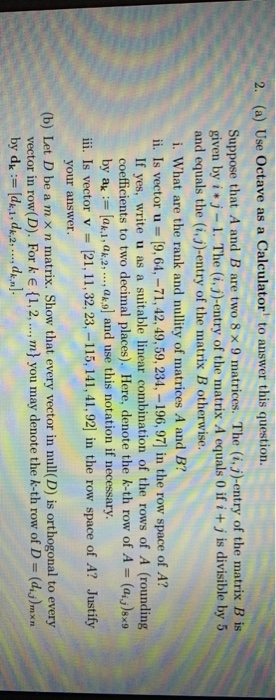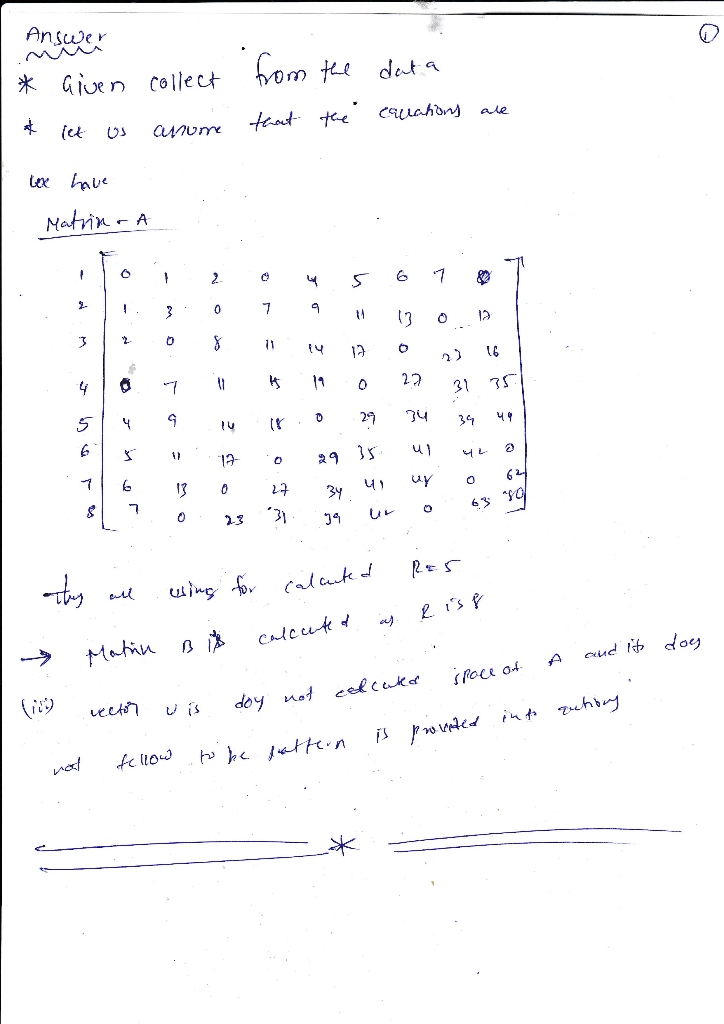Homework Help Question & Answers

2. (a) Use Octave as a Calculator to answer this question Suppose that A and B are two 8 x 9 matrices. The (i, j)-entry of the matrix B is given by i j-1. The (i.j)-entry of the matrix A equals 0...2. (a) Use Octave as a Calculator to answer this question Suppose that A and B are two 8 x 9 matrices. The (i, j)-entry of the matrix B is given by i j-1. The (i.j)-entry of the matrix A equals 0 if i +j is divisible by 5 and equals the (i,j)-entry of the matrix B otherwise. i. What are the rank and nullity of matrices A and B? i. Is vector u 9,64, -71,42, 49,59, 234,-196,97] in the row space of A? If yes, write u as a suitable linear combination of the rows of A (rounding coefficients to two decimal places). Here, denote the k-th row of A (aj)sx9 by a la1, ak.,, a and use this notation if ne iii. Is vector v = [21, 11, 32, 23,-115,141, 41,92 in the row space of A? Justify your answer. (b) Let D be a m x n matrix. Show that every vector in null(D) is orthogonal to every vector in row(D). For k E (1,2,.., m you may denote the k-th row of D- (d)mxn by d dk.i,d].Add Answer of: 2. (a) Use Octave as a Calculator to answer this question Suppose that A and B are two 8 x 9 matrices. The (i, j)-entry of the matrix B is given by i j-1. The (i.j)-entry of the matrix A equals 0...
More Homework Help Questions Additional questions in this topic.

• 1/4x-1/6y=-2 -1/6x+1/5y=4 solve for x and y its suppose to be x equals 12 and y equals 30 need steps thanks

Need Online Homework Help?

Get FREE EXPERT Answers
WITHIN MINUTES
Related Questions